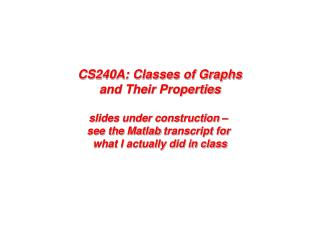# Some classes of graphs - PowerPoint PPT PresentationDownload PresentationSome classes of graphs

Some classes of graphsDownload Presentation## Some classes of graphs

- - - - - - - - - - - - - - - - - - - - - - - - - - - E N D - - - - - - - - - - - - - - - - - - - - - - - - - - -
##### Presentation Transcript

1. CS240A: Classes of Graphsand Their Propertiesslides under construction – see the Matlab transcript for what I actually did in class

2. Some classes of graphs • Classifications of graphs: • Planar graphs (drawable without crossing edges) • Overlap graphs (physical locality) • Power-law graphs (hist(vtx degree d) ~ d-β for some β > 0) • Small-world graphs (small diameter, large cluster coefficient) • Generators for classes of graphs: • Erdos-Renyi (flat) random graphs: sprandsym.m • RMAT random graph generator: rmat.m • 2-D and 3-D mesh generators: grid5.m etc. in meshparttoolbox • Graphs observed in the wild (see Florida collection for many examples): • Finite element meshes: meshes.m • Circuit simulation graphs: circuit_3.mat • Relationship networks: coAuthorsDBLP.mat, PGPgiantcompo.mat • … many others!

3. Planar graphs

4. Overlap Graphs [Miller, Teng, Thurston, Vavasis] • A k-ply neighborhood system in d dimensions is a set {D1,…,Dn} of closed disks in Rd such that no point in Rd is interior to more than k disks • An (a,k) overlap graph (for a>= 1) has vertices at the centers of the disks {D1,…,Dn} of a k-ply neighborhood system, with an edge (i, j) if expanding the smaller disk (Di or Dj) by amakes them overlap An n-by-n mesh is a (1,1) overlap graph Every planar graph is (a,k) overlap 2D Mesh is (1,1) overlap graph

5. RMAT Approximate Power-Law Graph

6. Strongly connected components of an RMAT graph

7. Diversity of graphs in the wild… Low diameter graph (R-MAT) vs. Long skinny graph (genomics) Gene linkage map, courtesy Yan et al.

8. Some graph statistics (and Matlab tools) • Vertex degree histogram: dhist.m • BFS level profile, gives a feeling for avg shortest paths : bfslevels.m • Clustering coefficient: ccoeff.m • c = 3*(# triangles) / (# connected triples) • Laplacian eigenvalues (and vectors): meshparttoolbox, eigs(laplacian(A),5,’lm’) • Separator size: meshpart toolbox • Fill (chordal completion size): analyze.m and amd.m